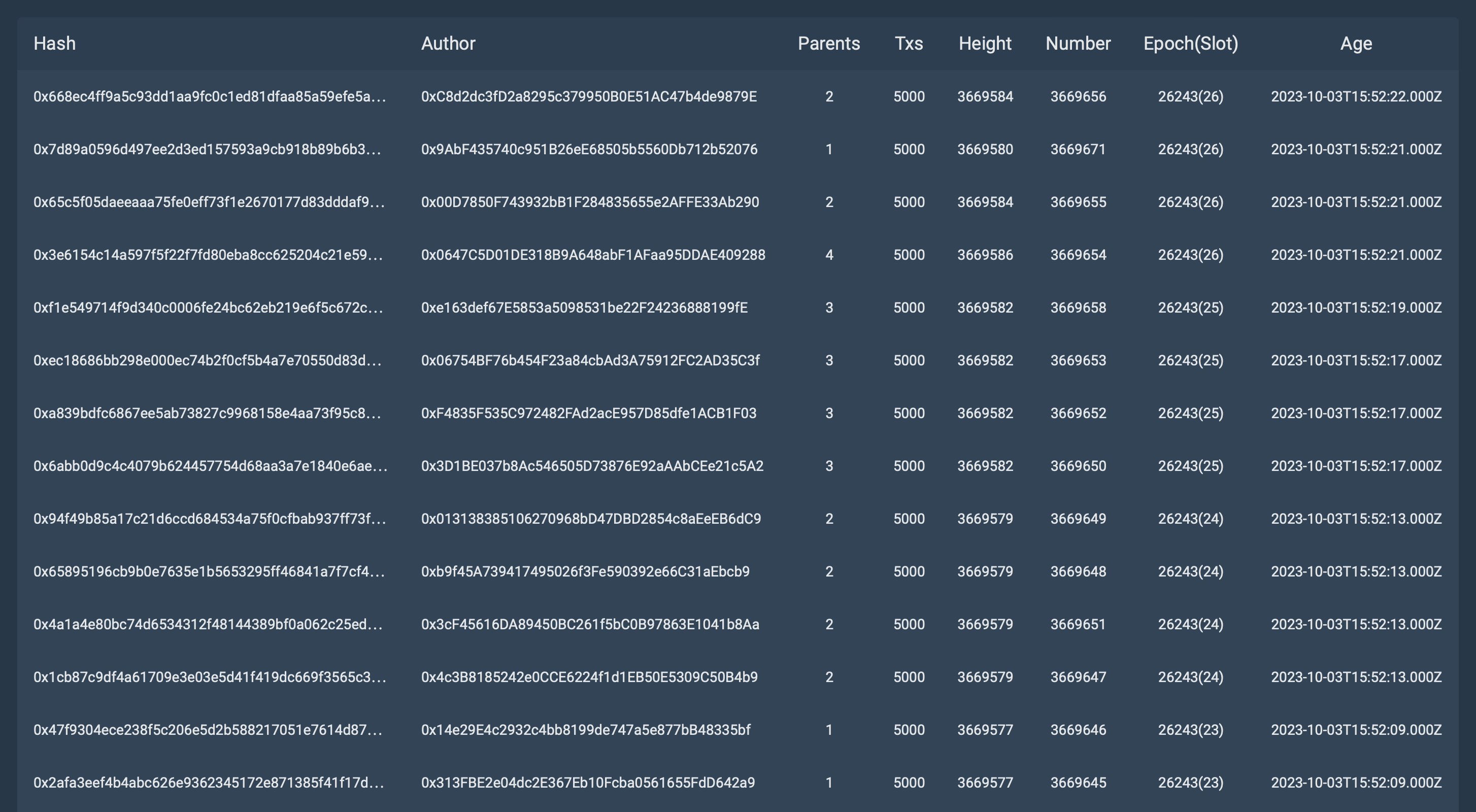# Testnet 7 Test 11

For this test, we used Digital Ocean Basic Droplet with the following server characteristics:

• Name: `s-2vcpu-4gb`
• vCPUs: `2`
• Memory (GiB): `4.0`
• SSD Disk (GiB): `80.0`
• Network Transfer (TB): `4.0`

Network settings:

• Slot duration: `4 sec`
• Nodes: `64`
• Workers: `32 per node`

Algorithm of actions during testing:

1. Stop creating blocks in the tested network.
2. Generates ~70,000 transactions.
3. Start 64 nodes.
4. Fix the time in which all these transactions were added and accepted into blocks, i.e. executed.

Let’s analyze the line from the test results below:

Block number: 3669645
Number of transactions: 5000
Age(slot): 26243(23)

Block number: 3669646
Number of transactions: 5000
Age(slot): 26243(23)

Block number: 3669647
Number of transactions: 5000
Age(slot): 26243(24)

Block number: 3669648
Number of transactions: 5000
Age(slot): 26243(24)

Block number: 3669649
Number of transactions: 5000
Age(slot): 26243(24)

Block number: 3669651
Number of transactions: 5000
Age(slot): 26243(24)

Block number: 3669650
Number of transactions: 5000
Age(slot): 26243(25)

Block number: 3669652
Number of transactions: 5000
Age(slot): 26243(25)

Block number: 3669653
Number of transactions: 5000
Age(slot): 26243(25)

Block number: 3669658
Number of transactions: 5000
Age(slot): 26243(25)

Block number: 3669654
Number of transactions: 5000
Age(slot): 26243(26)

Block number: 3669655
Number of transactions: 5000
Age(slot): 26243(26)

Block number: 3669656
Number of transactions: 5000
Age(slot): 26243(26)

Block number: 3669671
Number of transactions: 5000
Age(slot): 26243(26)

Let’s check how many transactions were added and processed in the two slots.
Take 3 slots = 12 seconds.
26243(24)-26243(26)

(5000+5000+5000+5000 + 5000+5000+5000+5000 + 5000+5000+5000+5000 ) = 60000

60000/12=5000 TPS.

Now let’s check the values for four slots.
If we take the 4th slot (26243(23)) (we can’t start block creation on all nodes simultaneously).
(60000 + 5000+5000) = 70000

70000/16=4375 TPS.